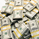1655 vues
1655
Shows the average from the last 5 periods for close price cycle. For example to see the annual seasonality of a stock for the last 5 years use on daily chart with the default setting of 252, the number of trading days in a year, approximately.
study("5 Period Cycle Seasonality")

Period = input(title="Period Cycle", type=integer , defval=252)

LastYear = close[Period]
TwoYearsAgo = close [2 * Period]
ThreeYearsAgo = close [3 * Period]
FourYearsAgo = close [4 *Period]
FiveYearsAgo = close [5 * Period]

Offset = Period
LastYearE = close[Period - Offset]
TwoYearsAgoE = close [2 * Period - Offset]
ThreeYearsAgoE = close [3 * Period - Offset]
FourYearsAgoE = close [4 *Period - Offset]
FiveYearsAgoE = close [5 * Period - Offset]

plot((LastYearE + TwoYearsAgoE + ThreeYearsAgoE + FourYearsAgoE + FiveYearsAgoE) / 5, color = #00ff00, linewidth = 5, offset =  Offset)

plot((LastYear + TwoYearsAgo + ThreeYearsAgo + FourYearsAgo + FiveYearsAgo) / 5, color = #ff0000, linewidth = 5)

Idées en relationGreat idea!

I've experimented with it some bit and find the following also interesting... It plots the average %-change instead of the average nominal change.

==

study("5 Period Cycle Seasonality")

Period = input(title="Period Cycle", type=integer , defval=252)

LastYear = (close-close)/close
TwoYearsAgo = (close -close)/close
ThreeYearsAgo = (close -close)/close
FourYearsAgo = (close -close)/close
FiveYearsAgo = (close -close)/close

Offset = Period
LastYearE = (close-close )/close
TwoYearsAgoE = (close -close )/close
ThreeYearsAgoE = (close -close )/close
FourYearsAgoE = (close -close )/close
FiveYearsAgoE = (close -close )/close

plot((LastYearE + TwoYearsAgoE + ThreeYearsAgoE + FourYearsAgoE + FiveYearsAgoE) / 5, color = #00ff00, linewidth = 2, offset = Offset)
plot((LastYear + TwoYearsAgo + ThreeYearsAgo + FourYearsAgo + FiveYearsAgo) / 5, color = #ff0000, linewidth = 2)
hline(0)

==
Répondreaccidentje
Seems TV can't handle code in the 'comment' field. I refer to
Répondre